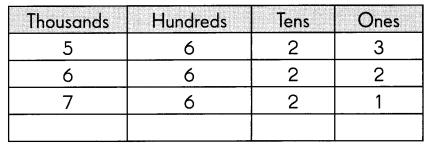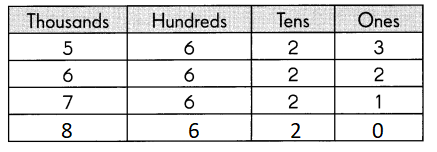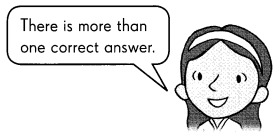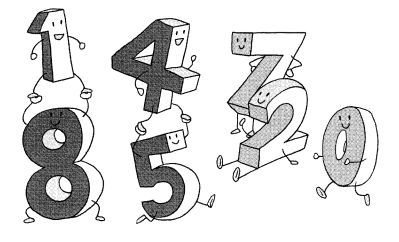# Math in Focus Grade 3 Chapter 1 Answer Key Numbers to 10,000

Go through the Math in Focus Grade 3 Workbook Answer Key Chapter 1 Numbers to 10,000 to finish your assignments.

## Math in Focus Grade 3 Chapter 1 Answer Key Numbers to 10,000

Challenging Practice

Write the missing number in the pattern.

Question 1.Complete each number pattern.
Explanation:
In thousands the numbers are increasing so the number is 8
5,6,7,8
In hundreds the number remains constant that is 6
In tens also the number remains constant that is 2
In ones the number is decreasing so the number is 0

Question 2.
5,621 5,741 5,861 ____
5,621 5,741 5,861 5,981
Explanation:
In the above pattern the place value of 100 is increasing 6 after 7
7 after 8 so, 8 after it should be 9
and the tens pattern is increasing by 2
2, 4 , 6 , 8

Question 3.
6,871 5,861 ___ 3,841
6,871 5,861  4851 3,841
Explanation:
In the above pattern the place value of 1000 is decreasing by 1 so, 6 after 5
5 after 4
and the tens pattern is decreasing by 1
7, 6 , 5 , 4

Question 4.
2,828 2,818 2,808 _____
2,828 2,818 2,808 2798
Explanation:
In the above pattern
The tens place is decreasing by 10
So the number should be
2798
2808 -10 = 2798

Fill in the mystery numbers.

Question 5.
I am a 3-digit number. The digit in my tens and ones places is the same. The digit in my hundreds place is 4 more than the digit in my tens and ones places.
I am ____Let us consider 2 will be the number is tens and ones place
4 more than tens and ones place is 2 + 4 = 6
If we choose 2 then 6 is the answer
if we choose the other number the answer will be changed.

Problem Solving

I am a 4-digit number.
The digit 8 is in the hundreds place.
The digit in the thousands place is greater than the digit in the hundreds place. The digit in the ones place is the smallest possible digit.
The digit in the tens place is 3 less than 6.
What number am I?
_________